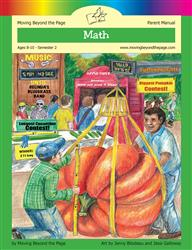Age 5-7 Reading Is Now Available!

# Common Core Alignment

CCSS.Math.Content.4.NF.4.b - Understand a multiple of a/b as a multiple of 1/b, and use this understanding to multiply a fraction by a whole number. For example, use a visual fraction model to express 3 × (2/5) as 6 × (1/5), recognizing this product as 6/5. (In general, n × (a/b) = (n × a)/b.)

## 5: MathUnit 5: Fractions
Lesson 12: Multiplying Fractions and Whole Numbers
Lesson 13: More Practice and Problem Solving
Final Project: Fraction BINGO